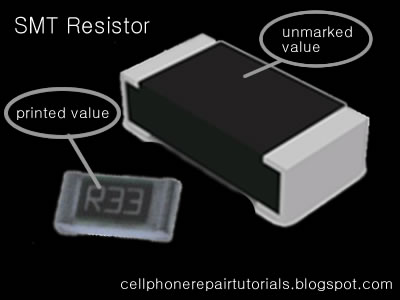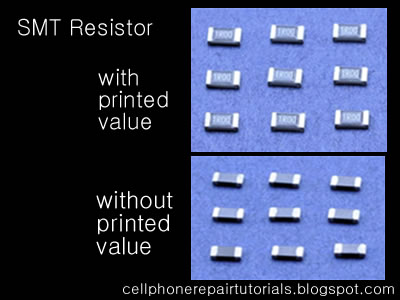GSM AMT

SMT ResistorSMT Resistor ( Unprinted ) In Mobile Phones Surface Mount Molded  (SMD) Resistor where not printed with numerical value and it is left blank, the problem is that it is too tiny or small to print at..
You can refer only its value by an aide of Schematic Diagram Available for that certain products. Or you can Identify and check its value by using Resistance Tester...In Schematic Diagram  Its Original value where indicated: For Example:

Resistances less than 1000 ohms or 1K  with ''R'' indicated in the middle indicates a decimal point like:

4R7 = 4.7Ω
2R2 = 2.2Ω

and the rest just like how it does indicated like:

100Ω  = 100  ohms
220Ω  = 200 ohms

and up
4.7K   = 4.7 kiloohms

The Printed SMD Resistor
Zero ohm resistors Surface mounted resistors are printed with numerical values in a code related to that used on axial resistors.Standard-tolerance Surface Mount Technology (SMT) resistors are marked with a three-digit code, in which the first two digits are the first two significant digits of the value and the third digit is the power of ten (the number of zeroes). For example:

334     = 33 × 10,000 Ω = 330 kΩ
222     = 22 × 100 Ω = 2.2 kΩ
473     = 47 × 1,000 Ω = 47 kΩ
105     = 10 × 100,000 Ω = 1 MΩ

Resistances less than 100 ohms are written: 100, 220, 470. The final zero represents ten to the power zero, which is 1. For example:

100     = 10 × 1 Ω = 10 Ω
220     = 22 × 1 Ω = 22 Ω

Resistances less than 10 ohms have 'R' to indicate the position of the decimal point (radix point). For example:

4R7     = 4.7 Ω
0R22     = 0.22 Ω
0R01     = 0.01 Ω

Precision resistors are marked with a four-digit code, in which the first three digits are the significant figures and the fourth is the power of ten. For example:

1001     = 100 × 10 ohms = 1 kΩ
4992     = 499 × 100 ohms = 49.9 kΩ
1000     = 100 × 1 ohm = 100 Ω

"000" and "0000" sometimes appear as values on surface-mount zero-ohm links, since these have (approximately) zero resistance.

Permissions in this forum:
You cannot reply to topics in this forum